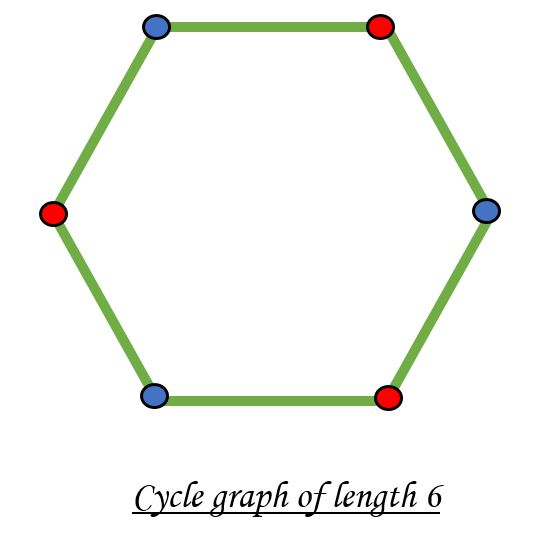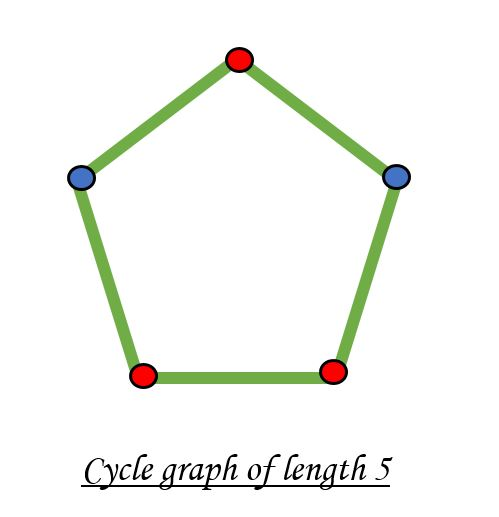# Check if a given graph is Bipartite using DFS

Given a connected graph, check if the graph is bipartite or not. A bipartite graph is possible if the graph coloring is possible using two colors such that vertices in a set are colored with the same color. Note that it is possible to color a cycle graph with even cycle using two colors. For example, see the following graph.It is not possible to color a cycle graph with an odd cycle using two colors.In the previous post, an approach using BFS has been discussed. In this post, an approach using DFS has been implemented. Given below is the algorithm to check for bipartiteness of a graph.

• Use a color[] array which stores 0 or 1 for every node which denotes opposite colors.
• Call the function DFS from any node.
• If the node u has not been visited previously, then assign !color[v] to color[u] and call DFS again to visit nodes connected to u.
• If at any point, color[u] is equal to !color[v], then the node is bipartite.
• Modify the DFS function such that it returns a boolean value at the end.

Below is the implementation of the above approach:

 `// C++program to check if a connected ` `// graph is bipartite or not suing DFS ` `#include ` `using` `namespace` `std; ` ` `  `// function to store the connected nodes ` `void` `addEdge(vector<``int``> adj[], ``int` `u, ``int` `v) ` `{ ` `    ``adj[u].push_back(v); ` `    ``adj[v].push_back(u); ` `} ` ` `  `// function to check whether a graph is bipartite or not ` `bool` `isBipartite(vector<``int``> adj[], ``int` `v, ` `                 ``vector<``bool``>& visited, vector<``int``>& color) ` `{ ` ` `  `    ``for` `(``int` `u : adj[v]) { ` ` `  `        ``// if vertex u is not explored before ` `        ``if` `(visited[u] == ``false``) { ` ` `  `            ``// mark present vertic as visited ` `            ``visited[u] = ``true``; ` ` `  `            ``// mark its color opposite to its parent ` `            ``color[u] = !color[v]; ` ` `  `            ``// if the subtree rooted at vertex v is not bipartite ` `            ``if` `(!isBipartite(adj, u, visited, color)) ` `                ``return` `false``; ` `        ``} ` ` `  `        ``// if two adjacent are colored with same color then ` `        ``// the graph is not bipartite ` `        ``else` `if` `(color[u] == color[v]) ` `            ``return` `false``; ` `    ``} ` `    ``return` `true``; ` `} ` ` `  `// Driver Code ` `int` `main() ` `{ ` `    ``// no of nodes ` `    ``int` `N = 6; ` ` `  `    ``// to maintain the adjacency list of graph ` `    ``vector<``int``> adj[N + 1]; ` ` `  `    ``// to keep a check on whether ` `    ``// a node is discovered or not ` `    ``vector<``bool``> visited(N + 1); ` ` `  `    ``// to color the vertices ` `    ``// of graph with 2 color ` `    ``vector<``int``> color(N + 1); ` ` `  `    ``// adding edges to the graph ` `    ``addEdge(adj, 1, 2); ` `    ``addEdge(adj, 2, 3); ` `    ``addEdge(adj, 3, 4); ` `    ``addEdge(adj, 4, 5); ` `    ``addEdge(adj, 5, 6); ` `    ``addEdge(adj, 6, 1); ` ` `  `    ``// marking the source node as visited ` `    ``visited = ``true``; ` ` `  `    ``// marking the source node with a color ` `    ``color = 0; ` ` `  `    ``// Function to check if the graph ` `    ``// is Bipartite or not ` `    ``if` `(isBipartite(adj, 1, visited, color)) { ` `        ``cout << ``"Graph is Bipartite"``; ` `    ``} ` `    ``else` `{ ` `        ``cout << ``"Graph is not Bipartite"``; ` `    ``} ` ` `  `    ``return` `0; ` `} `

Output:

```Graph is Bipartite
```

Time Complexity: O(N)
Auxiliary Space: O(N)

Attention reader! Don’t stop learning now. Get hold of all the important DSA concepts with the DSA Self Paced Course at a student-friendly price and become industry ready.

My Personal Notes arrow_drop_upCheck out this Author's contributed articles.

If you like GeeksforGeeks and would like to contribute, you can also write an article using contribute.geeksforgeeks.org or mail your article to contribute@geeksforgeeks.org. See your article appearing on the GeeksforGeeks main page and help other Geeks.

Please Improve this article if you find anything incorrect by clicking on the "Improve Article" button below.

Improved By : RishiAgrawal

Article Tags :
Practice Tags :

8

Please write to us at contribute@geeksforgeeks.org to report any issue with the above content.